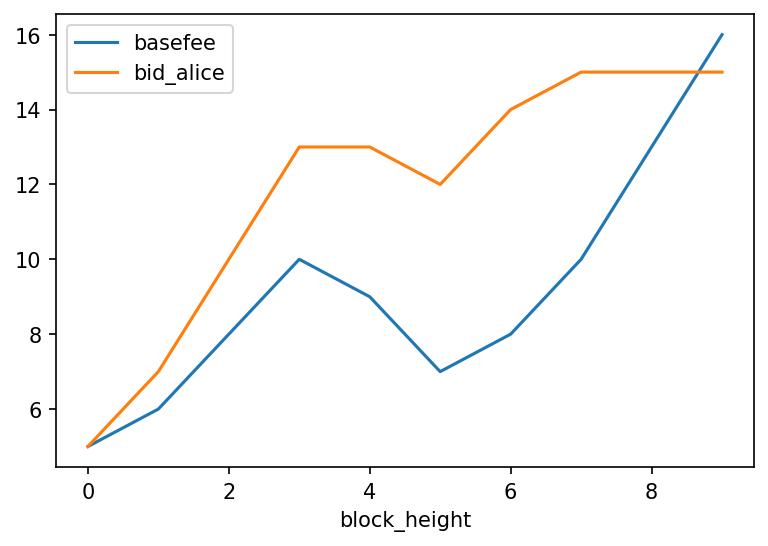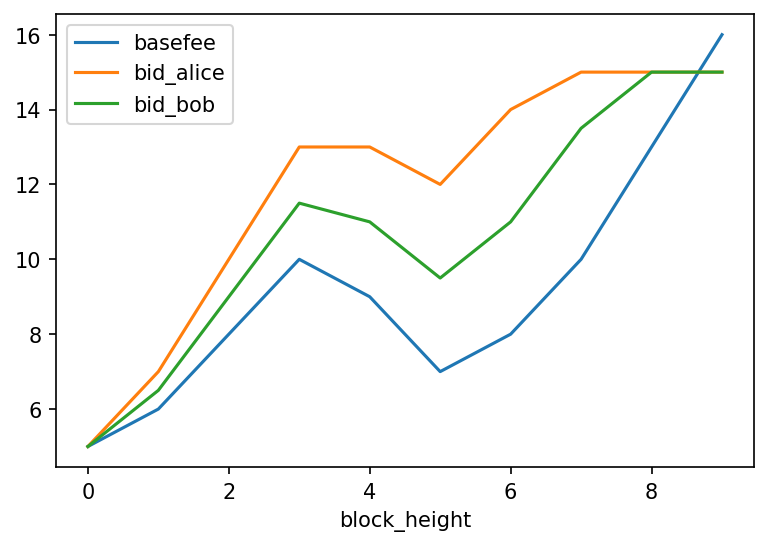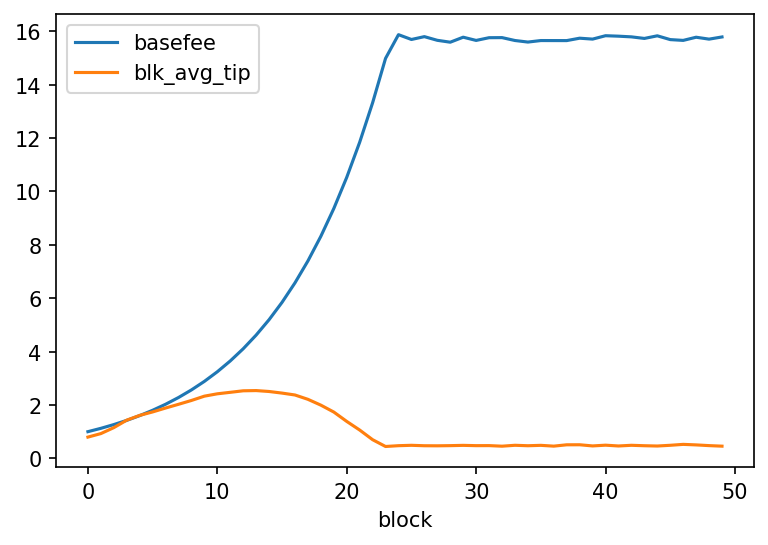## TL;DR¶

• We consider the floating escalator, a combination of 1559's "default price" and the escalator's "incremental bidding".
• We define and compare the efficiency of two user strategies:
• Hurrying users set the escalator slope to their cost for waiting one more unit of time, and balk whenever their current value is negative.
• Fixed duration users all set the length of the escalator to the same value, and set the slope to ramp as much as possible within that length.
• The two strategies give rise to different dynamics, which we interpret visually.
• While the social welfare is higher in hurry than in fixed, this may not matter as neither were shown to be equilibrium configurations. We focus instead on user efficiency, the total value realised by users in the market.
• We discuss optimality of fee markets and settle on a definition that seems natural for this setting. The user efficiency of both strategies is compared with this optimum, as well as a "random benchmark".

We've discussed EIP 1559 over the course of several notebooks. A competing proposal, dubbed "escalator", was introduced by Dan Finlay, taking inspiration from a paper by Agoric.

We take the current first-price auction paradigm as our benchmark. 1559 removes the auction component, attempting to quote a price for users based on supply of gas and demand for it. Users become price-takers most of the time, with their only decision being whether to transact or not.

The escalator proposal is a somewhat orthogonal direction from 1559. It retains some aspect of the first-price auction mechanism (users competing against each other) but allows users to "bump up" their bid following a linear formula. For instance, I initially bid 10 and specify that with each passing block, my bid should be increased by 5, until it reaches some maximum that I also defined. If I am included immediately, my gas price is 10. One block later, it is 15, etc.

The pattern of resubmitting transactions at a higher bid is known to most users of Ethereum. Manual resubmission of transactions is enabled by most wallets, while services such as any.sender allow you to programmatically emulate resubmission. The escalator automates it in protocol, in the sense that users do not need to manually resubmit, but set the parameters for the fee increase once and for all before sending the transaction to the pool, where its bid increases.

So on one axis we have a protocol-determined objective fee equalising supply and demand. On the other, we have control over bidding behaviour. The first is useful most of the time, in particular when demand is stationary. Yet the second may be desirable for these short periods where demand drastically changes and user behaviour reverts to strategic first-price auction-style bidding. Could we combine the two?

In this notebook, we investigate the floating escalator, a proposal to do so. We'll introduce its dynamics and study some user behaviours under this paradigm.

## Setting up¶

See this repository's README, for more instructions on how to run this notebook. The first step is to import the relevant objects from our library.

In :
import os, sys
sys.path.insert(1, os.path.realpath(os.path.pardir))
# You may remove the two lines above if you have installed abm1559 from pypi

from typing import Sequence

from abm1559.utils import (
constants,
)

from abm1559.txpool import TxPool

from abm1559.users import (
UserFloatingEsc,
)

from abm1559.userpool import UserPool

from abm1559.chain import (
Chain,
Block1559,
)

from abm1559.simulator import (
spawn_poisson_heterogeneous_demand,
update_basefee,
)

from abm1559.txs import (
Transaction,
TxFloatingEsc,
)

import pandas as pd
pd.set_option('display.max_rows', 20)
import numpy as np
import seaborn as sns

import matplotlib as mpl
mpl.rcParams['figure.dpi'] = 150


## Floating escalator 101¶

Since the floating escalator relies on a combination of both 1559 and the escalator, we'll introduce each one in turn before looking at their combination.

### Basefee in 1559¶

EIP 1559 targets a specific block size c. When blocks are too full, a price known as the basefee increases. More people want in? Fine, we'll raise the price. Over time, the basefee fluctuates, with higher values reached when more users want to transact.

The basefee is governed by a simple equation

basefee[t+1] = basefee[t] * (1 + d * (gas_used[t] - c) / c)


where gas_used[t] is the amount of gas used by block t. Note that blocks can use at most 2 * c gas, twice the target. When they do, and are full, the update rule above becomes basefee[t+1] = basefee[t] * (1 + d).

The adjustment speed d is currently set at 12.5%, which implies that the basefee after a full block increases by 12.5% (and basefee after an empty block decreases by 12.5%) .

### Escalator¶

In the escalator paradigm, a user submits:

• Their starting bid start_bid
• The starting block start_block
• How many blocks the bid is valid for, length
• Their maximum bid max_bid

Over length blocks, the true bid bid[t] at block t follows a linear interpolation of start_bid and max_bid, with

bid[t] = start_bid + (t - start_block) / length * (max_bid - start_bid)


At t = start_block, bid[t] = start_bid. At t = end_block, bid[t] = end_block.

In other words, the bid escalates slower or faster, depending on how much time it is valid for and how high the maximum bid is.

For example, if I submit 10 as my initial bid, 20 as my maximum, and 5 as the number of blocks over which my bid is valid for, then, as long as my transaction hasn't been included yet, my bid will increase by 2 every block for the next 5 blocks (in other words, until my bid reaches 20).

### Floating escalator¶

In the escalator paradigm, users must set a large number of parameters (start bid, max bid, duration of the escalator). Meanwhile, 1559 gives us this nice gas price "oracle" that tells you the market conditions as you start transacting. Combining the two would be nice! What if our escalator could "start" with the current 1559-predicated price, the basefee, and climb the bid from there?

What exactly climbs in the floating escalator? Remember that, under 1559, users specify a gas premium (or the maximum amount that a miner can receive from including the transaction). During transitions -- for example, a spike in demand -- we've seen users become strategic and "overbid". Ideally, there would exist some sort of fixed premium that would be expected to compensate the cost for a miner to include one extra transaction. The escalator governs the dynamics of this premium.

We call this hybrid "floating": we see the basefee as a kind of tide, rising and lowering with demand. The escalator starts at or near the tide, depending on start_premium. Meanwhile, the gas premium offered to the miners climbs in excess of the basefee. For instance, assume Alice starts her escalator at the basefee equal to 5 Gwei and increases the bid by 1 Gwei each block. She also specifies that she never wants to pay more than 15 Gwei.

In :
basefees = [5, 6, 8, 10, 9, 7, 8, 10, 13, 16]
bids_alice = [min(15, basefees[i] + i) for i in range(10)]

df = pd.DataFrame({
"block_height": range(10),
"basefee": basefees,
"bid_alice": bids_alice,
})
df.plot("block_height", ["basefee", "bid_alice"])

Out:
<AxesSubplot:xlabel='block_height'>Notice the distance between the basefee (in blue) and Alice's bids (in orange) increases over time, by 1 Gwei per block, until Alice reaches her maximum bid of 15.

At block 6, the basefee is 8 Gwei, so Alice's bid is 14 Gwei (8 Gwei from the basefee, 6 Gwei from her escalator). Then at block 7, the basefee increases to 10 Gwei. While Alice's bid ought to be 17 Gwei (10 Gwei from the basefee, 7 Gwei from her escalator), it is capped at 15 Gwei (the maximum amount Alice is willing to pay). We'll assume that after 10 blocks, Alice's transaction simply drops out.

Suppose a different user, Bob, starts at the same block as Alice, with an increase of 0.5 Gwei per block, and the same 15 Gwei limit.

In :
bids_bob = [min(15, basefees[i] + 0.5 * i) for i in range(10)]
df["bid_bob"] = bids_bob
df.plot("block_height", ["basefee", "bid_alice", "bid_bob"])

Out:
<AxesSubplot:xlabel='block_height'>We see Bob's bids in green. Notice that they keep below Alice's bids. In a sense, Bob is more conservative than Alice is. Alice might be in a hurry to get her bid included, and doesn't mind "overpaying" (i.e. taking the risk that the increment she chose was too large). Bob, on the other hand, prefers to slowly escalate his bid. All things equal, Alice should be included before Bob is, since miners receive the difference between her bid and the basefee.

## Hurried users in the floating escalator¶

In our model, users have both a value for the transaction, $v$, and a cost for waiting, $c$. Getting included immediately nets you a payoff of $v$, minus the transaction fees expended. Getting included 2 blocks later, $v - 2c$, minus the transaction fees, etc.

Since $c$ represents the time preferences of the user (a higher $c$ means it is more costly for me to wait), we could decide the escalator slope based on $c$: the higher the $c$, the higher the slope and escalator increments. This is an appropriate strategy for users who care about getting in as fast as possible given their waiting costs. For instance, users chasing an arbitrage opportunity or optimistic rollup dispute resolution transactions have high waiting costs, and thus would ramp up quickly within a short amount time.

Which brings us to the question: How long should the escalator ramp up for? Given bid increments of amount $s$, after $t$ blocks, assuming a constant basefee $b$, my bid is $b + t \times s$. Meanwhile, if my transaction is included at $t$, my payoff is $v - t \times c - (b + t \times s)$. We never want this payoff to become negative: since this would mean we would be worse off transacting than not! To ensure this never happens, we can figure out the number of blocks $t$ after which the previous expression becomes negative and use that as the duration of the escalator.

So how large should our increments be? We could set them to some fraction of the cost per unit, to respect the intuition that users who are in a hurry should set higher increments. To simplify for now, we'll set them to be exactly the user's cost per unit.

In our simulations, the users' bids won't start on the basefee exactly. We'll define the start_premium parameter as one escalator increment: if this increment is $c$, the first bid the user places is $b + c$ .

We've written a "dummy" UserFloatingEsc class in the library (abm1559/users.py) that we extend here to specify the parameters discussed above.

In :
class UserHurryEsc(UserFloatingEsc):

def decide_parameters(self, env):
basefee = env["basefee"]
slope = self.cost_per_unit
escalator_length = int(((self.value - basefee) / self.cost_per_unit - 1) / 2)
max_fee = basefee + (escalator_length + 1) * self.cost_per_unit
max_block = self.wakeup_block + escalator_length

tx_params = {
"max_fee": max_fee, # in wei
"start_block": self.wakeup_block,
"max_block": max_block,
"basefee": basefee,
}
return tx_params


Note that since the floating escalators have an expiry date (the max block after which they cannot be included), we create a new transaction pool which removes expired transactions.

In :
class TxPoolFloatingEsc(TxPool):

def add_txs(self, txs: Sequence[Transaction], env: dict) -> None:
invalid_txs = [tx_hash for tx_hash, tx in self.txs.items() if not tx.is_valid(env)]
self.remove_txs(invalid_txs)


As in our previous notebooks, we'll write out the main simulation loop.

In :
def simulate(demand_scenario, shares_scenario, TxPool=TxPoolFloatingEsc, rng=None):
# Instantiate a couple of things
txpool = TxPool()
basefee = constants["INITIAL_BASEFEE"]
chain = Chain()
metrics = []
user_pool = UserPool()

for t in range(len(demand_scenario)):

# env is the "environment" of the simulation
env = {
"basefee": basefee,
"current_block": t,
}

# We return some demand which on expectation yields demand_scenario[t] new users per round
users = spawn_poisson_heterogeneous_demand(t, demand_scenario[t], shares_scenario[t], rng=rng)

# Add users to the pool and check who wants to transact
# We query each new user with the current basefee value
# Users either return a transaction or None if they prefer to balk
decided_txs = user_pool.decide_transactions(users, env)

# New transactions are added to the transaction pool

# The best valid transactions are taken out of the pool for inclusion
selected_txs = txpool.select_transactions(env, user_pool=user_pool, rng=rng)
txpool.remove_txs([tx.tx_hash for tx in selected_txs])

# We create a block with these transactions
block = Block1559(txs = selected_txs, parent_hash = chain.current_head, height = t, basefee = basefee)

# The block is added to the chain

row_metrics = {
"block": t,
"basefee": basefee / (10 ** 9),
"users": len(users),
"decided_txs": len(decided_txs),
"included_txs": len(selected_txs),
"blk_avg_gas_price": block.average_gas_price(),
"blk_avg_tip": block.average_tip(),
"pool_length": txpool.pool_length,
}
metrics.append(row_metrics)

# Finally, basefee is updated and a new round starts
basefee = update_basefee(block, basefee)

return (pd.DataFrame(metrics), user_pool, chain)

In :
users_per_round = 2500
blocks = 50


We'll only simulate UserHurryEsc first, setting the average number of new users spawning between two blocks at 2,500. Our blocks can only accommodate 952 of them at most, so this will create congestion.

In :
rng = np.random.default_rng(42)
demand_scenario = [users_per_round for i in range(blocks)]

shares_scenario = [{
UserHurryEsc: 1,
} for i in range(blocks)]

(df_hurry, user_pool_hurry, chain_hurry) = simulate(demand_scenario, shares_scenario, rng=rng)


Let's observe some results!

In :
df_hurry

Out:
block basefee users decided_txs included_txs blk_avg_gas_price blk_avg_tip pool_length
0 0 1.000000 2542 2355 952 1.796545 0.796545 1403
1 1 1.124900 2473 2269 952 2.052800 0.927900 2684
2 2 1.265400 2515 2302 952 2.415915 1.150515 3907
3 3 1.423448 2437 2215 952 2.854479 1.431031 5015
4 4 1.601237 2430 2143 952 3.211069 1.609831 5968
... ... ... ... ... ... ... ... ...
45 45 15.687228 2520 469 469 16.176957 0.489729 0
46 46 15.657618 2508 505 505 16.181792 0.524174 0
47 47 15.776029 2426 459 459 16.280334 0.504306 0
48 48 15.704839 2479 496 496 16.182209 0.477370 0
49 49 15.786505 2464 460 460 16.243491 0.456987 0

50 rows × 8 columns

We recognise dynamics that should be familiar to us now. While the same average number of users spawn each block, and blocks are full in the first few steps, by the end of the simulation a much smaller number of users decides to actually transact (decided_txs). A new phenomenon is the pool_length being exactly zero by the end. Since transactions expire, old unincluded transactions are removed, while new transactions in the pool are all included. The basefee has reached its stationary level after which most users are priced out. This is confirmed by the following plot.

In :
df_hurry.plot("block", ["basefee", "blk_avg_tip"])

Out:
<AxesSubplot:xlabel='block'>Note the average tip in orange: in the first 20 blocks, when there is true competition from a shift in demand, many users want in given the low basefee amount, too many for all to be included. Those who wait in the pool see their bids escalate with increments equal to their cost per unit of waiting time. Miners of the blocks including highly escalated bids receive a much heftier tip, the difference between the user's bid and the current basefee. This comes to an end once basefee is stationary, after which priced out users do not even care to join the pool, escalating bids or not.

In :
# Obtain the pool of users (all users spawned by the simulation)
user_pool_hurry_df = user_pool_hurry.export().rename(columns={ "pub_key": "sender" })

# Export the trace of the chain, all transactions included in blocks
chain_hurry_df = chain_hurry.export()

# Join the two to associate transactions with their senders
user_txs_hurry_df = chain_hurry_df.join(user_pool_hurry_df.set_index("sender"), on="sender")

# We'll only look at the first 16 blocks
first_blocks = user_txs_hurry_df[user_txs_hurry_df.block_height <= 15].copy()
first_blocks["wakeup_block"] = first_blocks["wakeup_block"].astype("category")


Below, we are plotting the distribution of users included in each successive block. On the x-axis, we represent the value of the user $v_i$, while on the y-axis, we plot the cost per unit of time waiting $c_i$. Each point on one plot corresponds to one included transaction, with the point located at the (value, cost per unit) coordinates of the user. Additionally, we give a distinct colour to each wave of new users: users spawned before block 0 are blue, those spawned between blocks 0 and 1 are orange etc.

In :
g = sns.FacetGrid(data=first_blocks, col="block_height", col_wrap = 4)
g.map_dataframe(sns.scatterplot, x="value", y="cost_per_unit", hue="wakeup_block", palette="muted")